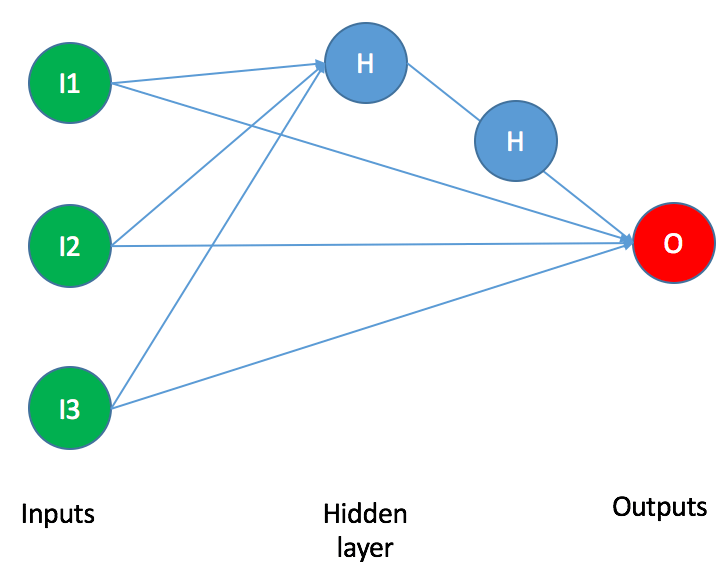Want to share your content on R-bloggers? click here if you have a blog, or here if you don't.

nnetsauce is now available to R users (currently, a development version). As a reminder, for those who are interested, the following page illustrates different use-cases for the nnetsauce, including deep learning application examples. This post from September 18 is about an Adaptive Boosting (boosting) algorithm variant available in the nnetsauce. This other post from September 25 presents a Bootstrap aggregating (bagging) algorithm variant also available in the nnetsauce, and is about recognizing tomatoes and apples.Not all Python functions are available in R so far, but the majority of them are. R implementation is catching up fast though. The general rule for invoking methods on objects in R as we’ll see in the example below, is to mirror the Python way, but replacing .’s by $‘s. Contributions/remarks are welcome as usual, and you can submit a pull request on Github. ## Installation Here is how to install nnetsauce from Github using R console: # use library devtools for installing packages from Github library(devtools) # install nnetsauce from Github devtools::install_github("thierrymoudiki/nnetsauce/R-package") # load nnetsauce library(nnetsauce)  Having installed and loaded nnetsauce, we can now showcase a simple classification example based on iris dataset and Ridge2Classifier model (cf. this paper and this notebook). With the iris dataset, we’d like to classify flowers as setosa, versicolor or virginica species, based on the following characteristics: sepal lengths, sepal widths, petal lengths and petal widths. ## Example Data preprocessing: library(datasets) # Explanatory variables: Sepal.Length, Sepal.Width, Petal.Length, Petal.Width X <- as.matrix(iris[, 1:4]) # Classes of flowers: setosa, versicolor, virginica y <- as.integer(iris[, 5]) - 1L # Number of examples n <- dim(X) # Split data into training/testing sets set.seed(123) index_train <- sample.int(n, size = floor(0.8*n)) X_train <- X[index_train, ] y_train <- y[index_train] X_test <- X[-index_train, ] y_test <- y[-index_train]  Fit Ridge2Classifier model to iris dataset with default parameters: # Create Ridge2Classifier model with 5 nodes in # the hidden layer, and clustering of inputs # all R-nnetsauce models are created this way obj <- Ridge2Classifier(activation_name='relu', n_hidden_features=5L, n_clusters=2L, type_clust='gmm') # Model fitting on training set # Notice Python .'s replaced by $'s
print(obj$fit(X_train, y_train))  Obtain model accuracy on test set: print(obj$score(X_test, y_test))


Obtain model probabilities on test set, for each class:

probs <- data.frame(obj\$predict_proba(X_test))
colnames(probs) <- dimnames(iris)[][1:3]


As a reminder, your examples of use of nnetsauce do have a home in this repository.

Note: I am currently looking for a gig. You can hire me on Malt or send me an email: thierry dot moudiki at pm dot me. I can do descriptive statistics, data preparation, feature engineering, model calibration, training and validation, and model outputs’ interpretation. I am fluent in Python, R, SQL, Microsoft Excel, Visual Basic (among others) and French. My résumé? Here!